# softmax激励函数

## softmax函数

softmax用于多分类过程中，它将多个神经元的输出，映射到（0,1）区间内，可以看成概率来理解，从而来进行多分类。

$S_i= \frac{ {e}^{V_i}}{\sum_{j}^{}{ {e}^{V_j}}}$

softmax直白来说就是将原来输出是3,1,-3通过softmax函数一作用，就映射成为(0,1)的值，而这些值的累和为1（满足概率的性质），那么我们就可以将它理解成概率，在最后选取输出结点的时候，我们就可以选取概率最大（也就是值对应最大的）结点，作为我们的预测目标！

## softmax相关求导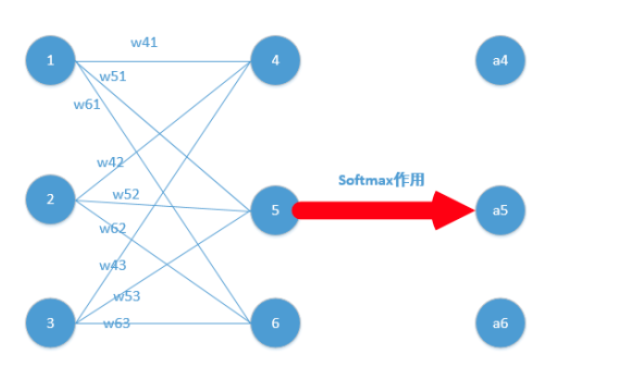我们能得到下面公式：

$z_{4} = w_{41} * o_{1} + w_{42} * o_{2} + w_{43} * o_{3} \\ z_{5} = w_{51} * o_{1} + w_{52} * o_{2} + w_{53} * o_{3} \\ z_{6} = w_{61} * o_{1} + w_{62} * o_{2} + w_{63} * o_{3}$

z4,z5,z6分别代表结点4,5,6的输出，01,02,03代表是结点1,2,3往后传的输入.

$a_4 = \frac{ {e}^{z_4}}{ {e}^{z_4}+{e}^{z_5}+{e}^{z_6}} \\ a_5 = \frac{ {e}^{z_5}}{ {e}^{z_4}+{e}^{z_5}+{e}^{z_6}} \\ a_6 = \frac{ {e}^{z_6}}{ {e}^{z_4}+{e}^{z_5}+{e}^{z_6}}$

$Loss = - \sum_{i}y_i ln_{a_i}$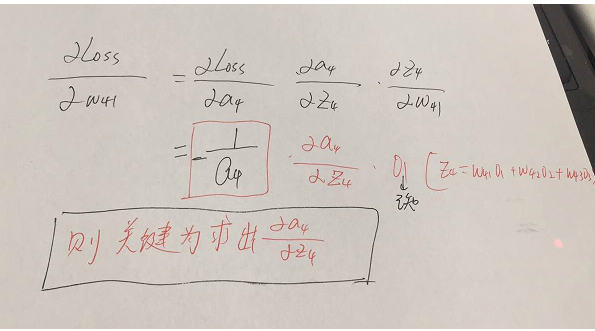w51…..w63等参数的偏导同理可以求出，那么我们的关键就在于Loss函数对于结点4,5,6的偏导怎么求，如下：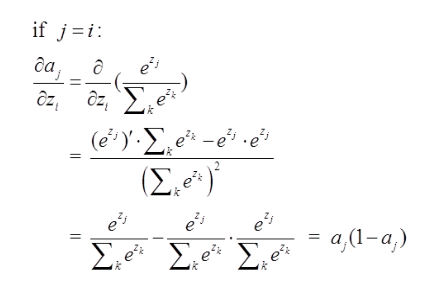j=i对应例子里就是如下图所示：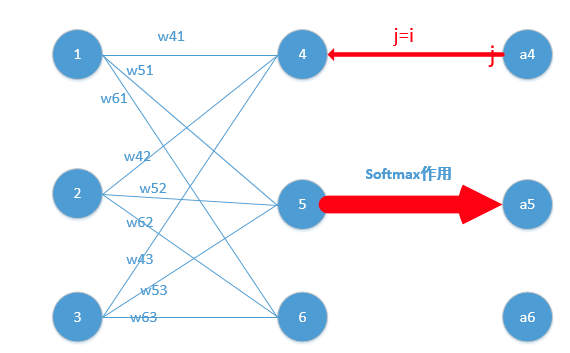$Loss=-lna_j，$他的导数是$-\frac{1}{a_j}$，与上面$a_j(1-a_j)$相乘为$a_j-1$。形式非常简单，这说明我只要正向求一次得出结果，然后反向传梯度的时候，只需要将它结果减1即可，后面还会举例子！）那么我们可以得到Loss对于4结点的偏导就求出了了（这里假定4是我们的预计输出）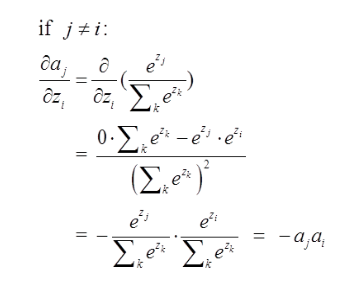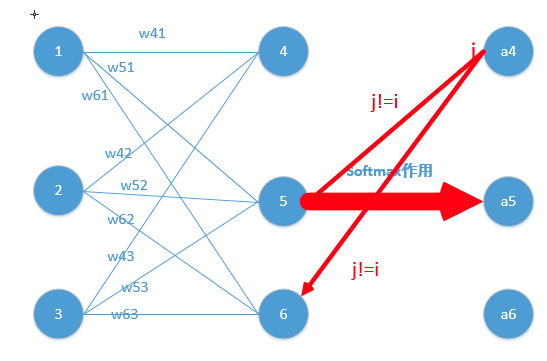$Loss=-lna_j$，他的导数是$-\frac{1}{a_j}$，与上面$-a_ja_i$相乘为$a_i$。（形式非常简单，这说明我只要正向求一次得出结果，然后反向传梯度的时候，只需要将它结果保存即可，后续例子会讲到）这里就求出了除4之外的其它所有结点的偏导，然后利用链式法则继续传递过去即可！我们的问题也就解决了！

$\begin{bmatrix} \frac{ {e}^{z_4}}{ {e}^{z_4}+{e}^{z_5}+{e}^{z_6}} & \frac{ {e}^{z_5}}{ {e}^{z_4}+{e}^{z_5}+{e}^{z_6}} & \frac{ {e}^{z_6}}{ {e}^{z_4}+{e}^{z_5}+{e}^{z_6}} \end{bmatrix}$ $\begin{bmatrix} 0.0903 & 0.2447 & 0.665 \end{bmatrix}$

$\begin{bmatrix} 0.0903 & 0.2447-1 & 0.665 \end{bmatrix}$